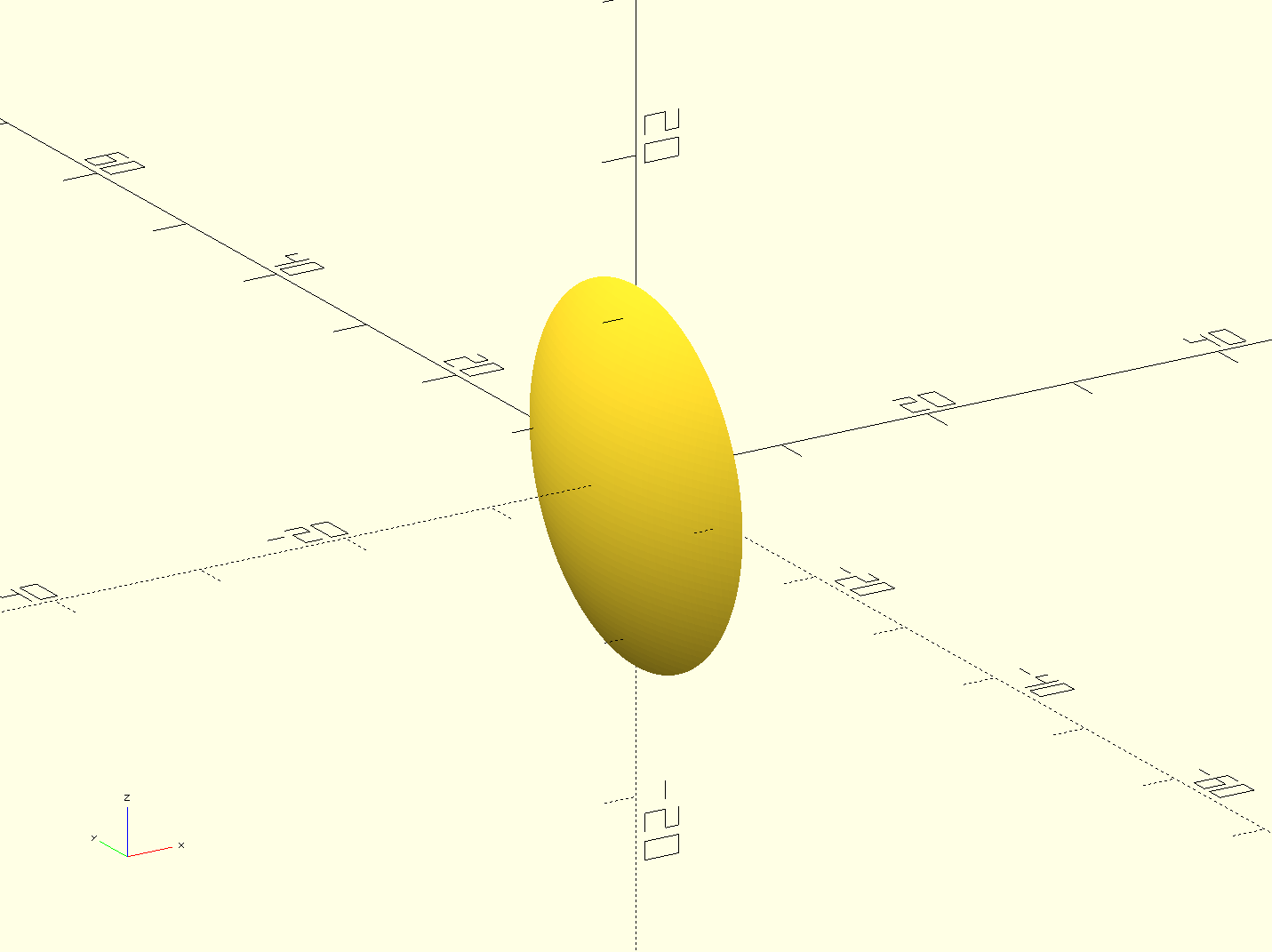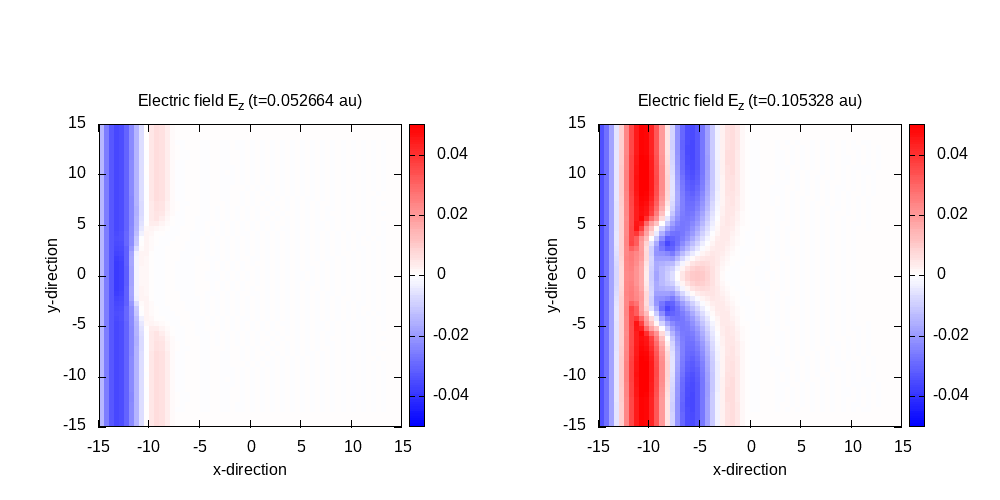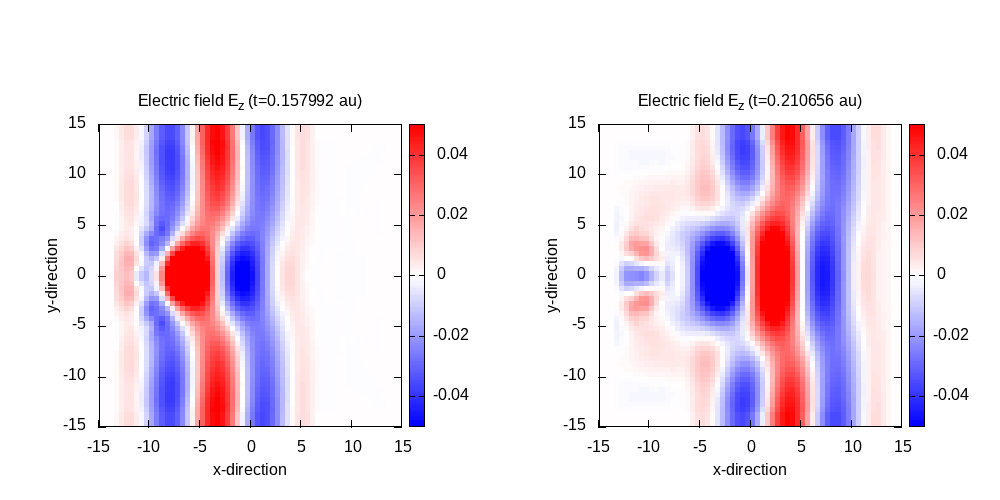# Non-dispersive linear media

## Including non-disperive linear media objects

An arbitrary number of linear medium shapes can be placed inside simulation box. Linear media are defined as a separate system type, for example:


%Systems
'Maxwell' | maxwell
'Medium'  | linear_medium
%


The object shape can be defined in two ways, which are given by the variable LinearMediumBoxShape: if set to medium_parallelepiped, the parallelepiped box will be defined by its center, and size in each dimension through the LinearMediumBoxSize block; if the box shape is defined as medium_box_file, the box shape will be read from an external geometry file in OFF format, defined through the variable LinearMediumBoxFile.

In this tutorial, we will simulate light propagation though a spherical lens, for which we need to provide an OFF file representing a suitable shape. There are many software packages around to generate such files, but we recommend openSCAD and ctmconv which is part of OpenCTM.

OpenSCAD provides its own scripting language for creating geometries, which can be created in a GUI, but also can be rendered on the command line. Here we will use the following code to create a simple lens from the intersection of two spheres and shift its position to the negative x-axis with openSCAD, using the following script:


$fs=2.0;$fa=2.0;
translate([-10,0,0]) {
intersection(){
translate([-8,0,0]) sphere(r=10);
translate([ 8,0,0]) sphere(r=10);
};
};


copy the above code into a file lens.scad, you can generate the required OFF file by the command:

openscad -o lens.off lens.scad


For details on the syntax of this, see the openSCAD tutorials.
This creates the following lens:Now we have the OFF file, let’s consider the electromagnetic properties of the medium. These must be defined in the LinearMediumProperties block, which specifies the relative electric permittivity, relative magnetic permeability, and the electric and magnetic conductivities (for lossy media). In addition to adding the linear_medium system, we need to switch the MaxwellHamiltonianOperator to faraday_ampere_medium.

As a linear medium will scatter waves in all directions, there need to be absorbing boundary conditions to avoid spurious reflections on the box boundaries. For this purpose, we change the MaxwellAbsorbingBoundaries block to mask in all directions, to damp the field at the boundaries by multiplying it with a scalar mask function. The width of this mask MaxwellABMaskWidth is set to 5 Bohr.

As a consequence of the additional region for the absorbing boundary condition, we have to increase the box size. Therefore, the lsize_mx value is now 20.0 (13.0 Bohr for propagation region plus 2.0 Bohr for the incident wave boundaries plus 5.0 Bohr for absorbing boundary conditions).

Finally, as non-dispersive linear media are static, TDSystemPropagator = static , which is the default option, must be set for the medium, while the Maxwell system needs to be set to exp_mid. A possible input file to run this simulation, then, would be the following:


CalculationMode = td
ExperimentalFeatures = yes

%Systems
'Maxwell' | maxwell
'Medium'  | linear_medium
%

Maxwell.ParDomains = auto
Maxwell.ParStates  = no

# free maxwell box limit of 13.0 plus 2.0 for the incident wave boundaries with
# der_order times dx_mx (here: der_order = 4) plus 5.0 for absorbing boundary conditions

lsize_mx = 20.0
dx_mx    = 0.5

BoxShape = parallelepiped

%Lsize
lsize_mx | lsize_mx | lsize_mx
%

%Spacing
dx_mx | dx_mx | dx_mx
%

LinearMediumBoxShape = medium_box_file
LinearMediumBoxFile = "lens.off"
%LinearMediumProperties
5.0 | 1.0 | 0.0 | 0.0
%

%MaxwellBoundaryConditions
plane_waves | plane_waves | plane_waves
%

%MaxwellAbsorbingBoundaries
%

MaxwellABWidth = 5.0

Maxwell.TDSystemPropagator = exp_mid

timestep = 1 / ( sqrt(c^2/dx_mx^2 + c^2/dx_mx^2 + c^2/dx_mx^2) )
Maxwell.TDTimeStep = timestep
Medium.TDTimeStep = timestep/2
TDPropagationTime = 150*timestep

OutputFormat = plane_x + plane_y + plane_z + axis_x + axis_y + axis_z

%MaxwellOutput
electric_field
%

MaxwellOutputInterval = 25
MaxwellTDOutput = maxwell_energy + maxwell_total_e_field + maxwell_total_b_field

lambda1 = 10.0
omega1  = 2 * pi * c / lambda1
k1_x    = omega1 / c
E1_z    = 0.05
pw1     = 10.0
ps1_x   = - 25.0

# laser propagates in x direction
%MaxwellIncidentWaves
plane_wave_mx_function | 0 | 0 | E1_z | "plane_waves_function_1"
%

%MaxwellFunctions
"plane_waves_function_1" | mxf_cosinoidal_wave | k1_x | 0    | 0 | ps1_x | 0     | 0 | pw1
%


For this run, we use the previous incident plane wave propagating only in the x-direction but place a medium box inside the simulation box. After running the code, we can visualize the results with the following gnuplot script:

gnuplot script

Contour plot of the electric field in z-direction after 50 time steps for t=0.11 and 100 time steps for t=0.21:Contour plot of the electric field in z-direction after 125 time steps for t=0.26 and 150 time steps for t=0.32:In the last panel, it can be seen that there is a significant amount of scattered waves which, in large parts, are scattered from the box boundaries. This is due to making use of plane wave boundary conditions and absorbing boundaries simultaneously, and would be less noticeable if we increase the size of the box. In this tutorial we keep the box small to stay within a reasonable computation time.